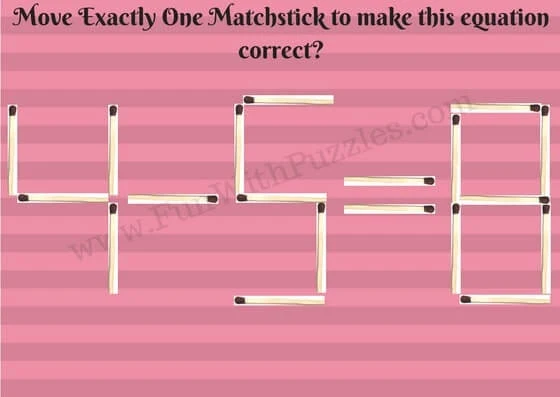In this matchstick Math picture puzzle, one mathematical equation is given. This mathematical equation is created using matchsticks. This equation is not correct. However, this equation can be corrected using exactly one matchstick. Your challenge is to move exactly one matchstick and correct this Mathematical Equation.Move Exactly One Matchstick to make this equation correct.

The answer to this "Quick Matchstick Maths Picture Puzzle" can be viewed by clicking the button. Please do give your best try before looking at the answer.

## List of similar Maths and Logic Brain Teasers

Please check out the following puzzles and brain teasers to continue challenging your mind.

1. Matchstick Maths Picture Puzzles for Kids: Easy matchstick puzzles in which one has to move exactly one matchstick to correct the given Mathematical Equation.

2. Picture Matchstick Puzzles: These are the visual Matchstick Puzzles to test your observational skills.

3. Easy Mathematical Brain Teasers with Answers: It contains many easy Mathematical brain teasers to twist your mind.